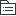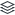# 项目整合管理

201
|
2

## 相关模板推荐

•## 项目整合管理 —— 作品大纲

•启动
• 制定项目章程
• 内容
• 高层级的需求
• 项目退出标准
• 可测量的项目目标和相关的成功标准
• 整体项目风险
• 总体里程碑进度计划
• 高层级项目描述，边界定义以及主要可交付成果
• 关键相关方名单
• 委派的项目经理及其权责和职权
• 发起人或其他批准项目章程的人员姓名和职权
• 项目审批要求
• 预先批准的财务资源
• 作用
• 明确项目与组织战略目标之间的直接联系
• 高级管理层明确表示是他们对项目的支持
• 展示组织对项目的承诺
• 体现项目的优先级
• 描述与项目集/项目组合的关系
• 确立项目的正式地位
• 授权项目经理
• 授权项目经理规划，执行和控制项目
• 初步确定项目的高层级需求，目标
• 初步确定项目的关键假设和约束
• 在执行组织与需求组织之间简历起伙伴关系
• 过程
• 输入
• 商业文件
• 商业论证
• 效益管理计划
• 协议
• 事业环境因素
• 组织过程资产
• 工具
• 专家判断
• 数据收集
• 头脑风暴
• 焦点小组
• 访谈
• 人际关系与团队技能
• 冲突管理
• 引导
• 会议管理
• 会议
• 输出
• 项目章程
• 假设日志
•规划
• 制定项目管理计划
• 定义
• 定义，准备和协调项目计划的所有组成部分，并把他们整合为一份综合项目管理计划的过程
• 作用
• 生成一份综合文件，用于确定所以项目工作的基础及其执行方式，仅开展一次或在项目的预定义点开展
• 过程
• 输入
• 项目章程
• 其他过程的输出
• 事业环境因素
• 组织过程资产
• 工具
• 专家判断
• 数据收集
• 人际关系与团队技能
• 会议
• 输出
• 项目管理计划
•执行
• 指导与管理项目文件
• 定义
• 为实现项目目标而领导和执行项目管理计划中所确定的工作，并实施已批准变更的过程。
• 作用
• 对项目工作和可交付成果开展综合管理，以提高项目成功的可能性。
• 过程
• 输入
• 项目管理计划
• 项目文件
• 变更日志
• 经验教训登记册
• 里程碑清单
• 项目沟通记录
• 项目进度计划
• 需求跟踪矩阵
• 风险登记册
• 风险报告
• 批准的变更请求
• 事业环境因素
• 组织过程资产
• 工具
• 专家判断
• 项目管理信息系统PMIS
• 会议
• 输出
• 可交付成果
• 工作绩效数据
• 问题日志
• 变更请求
• 纠正措施
• 预防措施
• 缺陷补救
• 更新
• 项目管理计划更新
• 项目文件更新
• 活动清单
• 日志
• 需求文件
• 风险登记册
• 相关方登记册
• 组织过程资产更新
• 管理项目知识
• 定义
• 使用现有知识并生成新知识，以实现项目目标，并且帮助组织学习的过程。
• 作用
• 利用已有的组织知识来创造或该井项目成果，并且使用当前项目创造的知识可用于支持组织运营和未来的项目或阶段。
• 过程
• 输入
• 项目管理计划
• 项目文件
• 经验教训登记册
• 项目团队派工单
• 资源分解结构
• 供方选择标准
• 相关方登记册
• 可交付成果
• 事业环境因素
• 组织过程资产
• 工具
• 专家判断
• 知识管理
• 信息管理
• 人际关系与团队技能
• 输出
• 经验教训登记册
• 项目管理计划更新
• 组织过程资产更新
•监控
• 监控项目工作
• 定义
• 跟踪，审查和报告整体项目进展，以实现项目管理计划中确定的绩效目标的过程
• 作用
• 让相关方了解项目的当前状态并认可为处理绩效哦啊问题而采取的行动，以及通过成本和进度预测，让相关方了解未来项目的状态。
• 过程
• 输入
• 项目管理计划
• 项目文件
• 假设日志
• 估算依据
• 成本预测
• 问题日志
• 经验教训登记册
• 里程碑清单
• 质量报告
• 风险登记册
• 风险报告
• 进度预测
• 工作绩效信息
• 协议
• 事业环境因素
• 组织过程资产
• 工具
• 专家判断
• 数据分析
• 备选方案分析
• 成本效益分析
• 挣值分析
• 根本原因分析
• 趋势分析
• 偏差分析
• 决策
• 会议
• 输出
• 工作绩效报告
• 变更请求
• 项目管理计划更新
• 项目文件更新
• 成本预测
• 问题日志
• 经验教训登记册
• 风险登记册
• 进度预测
• 实施整体变更控制
• 定义
• 审查所以变更请求，批准变更，该你了对可交付成果，项目文件和项目该你了计划的变更，并对变更处理结果进行沟通的过程。
• 作用
• 确保对项目中记录在案的变更做综合评审
• 过程
• 输入
• 项目管理计划
• 变更管理计划
• 配置管理计划
• 范围基准
• 进度基准
• 成本基准
• 项目文件
• 估算依据
• 需求跟踪矩阵
• 风险报告
• 工作绩效报告
• 变更请求
• 事业环境因素
• 组织过程资产
• 工具
• 专家判断
• 变更控制工具
• 数据分析
• 备选方案分析
• 成本效益分析
• 决策
• 投票
• 独裁型决策制定
• 多标准决策分析
• 会议
• 输出
• 批准的变更请求
• 变更请求
• 项目管理计划更新
• 项目文件更新
• 变更日志
•收尾
• 结束项目或阶段
• 定义
• 终结项目，阶段或合同的所有活动的过程。
• 作用
• 存档项目或阶段信息，完成计划的工作，释放组织团队资源以开展新的工作。
• 过程
• 输入
• 项目章程
• 项目管理计划
• 项目文件
• 假设日志
• 估算依据
• 变更日志
• 问题日志
• 经验教训登记册
• 里程碑清单
• 项目沟通记录
• 质量控制测量报告
• 质量报告
• 风险登记册
• 验收的可交付成果
• 商业文件
• 商业论证
• 效益管理计划
• 协议
• 采购文档
• 组织过程资产
• 工具
• 专家判断
• 数据分析
• 文件分析
• 回归分析
• 趋势分析
• 偏差分析
• 会议
• 输出
• 项目文件更新
• 最终产品，服务或成果移交
• 最终报告
• 组织过程资产更新